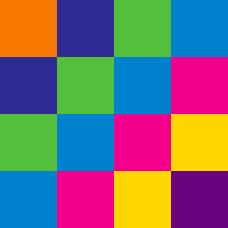Everyday Math

# Pattern Recognition: Level 1 Challenges

Assuming the pattern below continues, how many squares will be in Figure 5?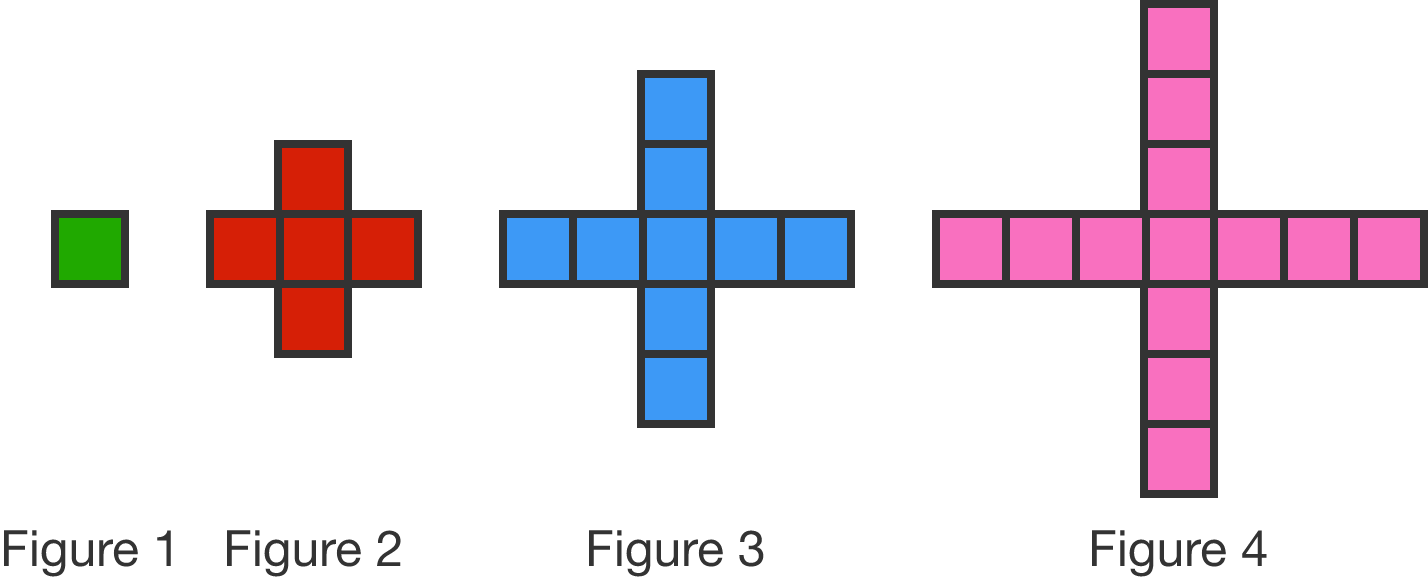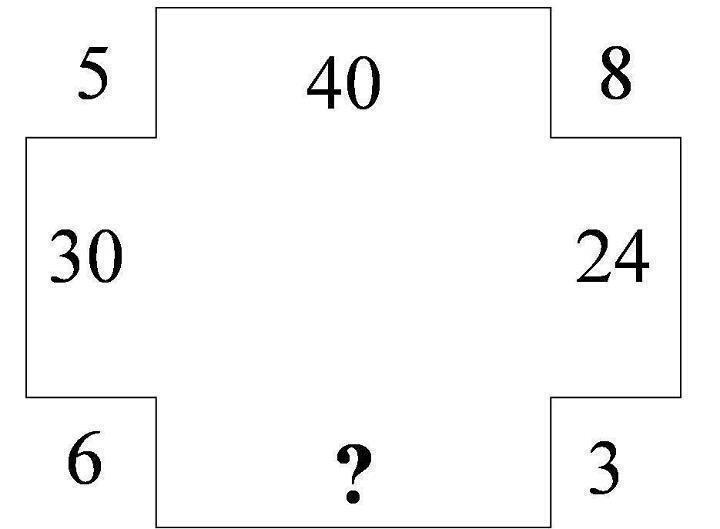What number fits into the question mark?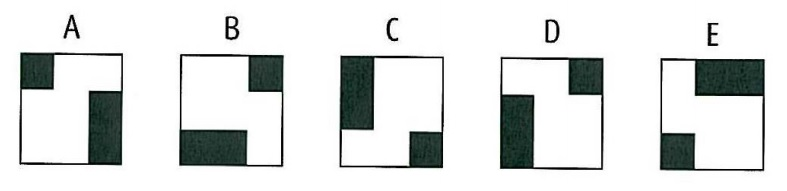Which shape is the odd one out?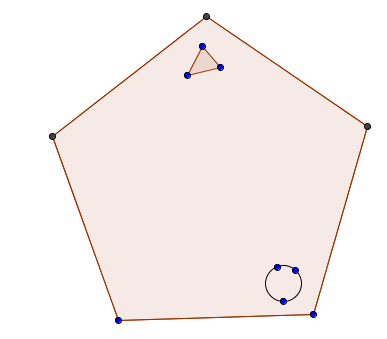The circle moves two places anti-clockwise at each stage and the triangle moves one place clockwise at each stage. After how many stages will they be together in the same corner?

Note : Moving one places signifies moving one corner.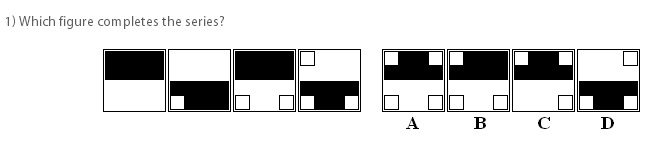Which figure completes the series?

×